Next: Signed Binary Stream Operations Up: Dyadic Stream Operations Previous: Average

## Multiplication

Multiplying two streams of dyadic digits is also a relatively simple operation, Suppose we wish to multiply two streams of the form (a::x) and (b::y). Using the properties described in section 3.6.2 we can say

From this we can now obtain a simple recursive algorithm: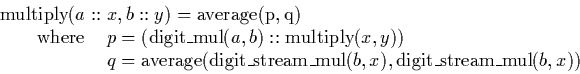This uses the operations to average two streams (section 4.2.1), multiply two digits (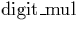, see section A.3), multiply a stream by a digit (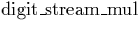, see sections 4.1.2 and A.3), and a recursive call to itself to multiply two streams.

Observe that the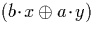term is directly computable from the input, we know one digit or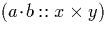without needing to make a recursive call to this dyadic stream multiplication algorithm, and the dyadic stream average operation requires only one digit of each input to generate one digit of output. Hence an output digit is can be determined without making a recursive call and thus depends on a finite portion of the input. This argument also applies to the recursive call, and so it can be seen that this method is indeed convergent and we have an algorithm.

Martin Escardo
5/11/2000